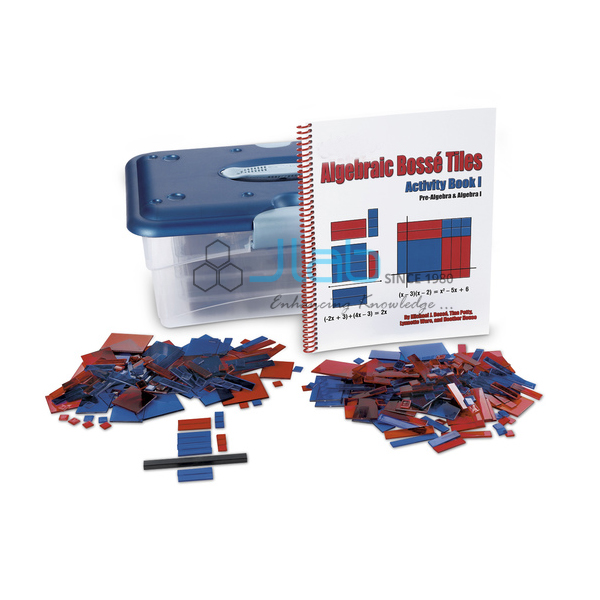# Algebraic Bosse Tiles X and YProduct Code : JL-MLI-9808

Algebraic Bosse Tiles X and Y
A visual aid to help reinforce and clarify algebraic principles by combining the power of Algebra Tiles and Algebra Lab Gear.
Bosse Tiles are manipulatives that represent the different elements of an algebraic equation.
Using these manipulatives allows students to make a hands-on connection to algebraic concepts.
Tiles can represent the product of linear polynomials up to three variables or the factoring of quadratic polynomials with as many as six terms.
Tiles are translucent, allowing for use on an overhead.
The Bosse Tiles books provide teachers and students with a variety of practice problems and information about using the Bosse Tiles to find and double-check answers.
Set includes 4 sets of the x and y Bosse Tiles.